# Internal variations, method of

A method in the theory of functions of a complex variable which is employed in solving extremal problems in classes of univalent and multivalent analytic functions. The advantages of the method of internal variations consist in the fact that in choosing the variational formulas on which it is based no assumptions are made on the behaviour of the functions being varied on the boundary of their domain of definition, while the variational formulas themselves are a consequence of variations (modifications) of the functions in the class in a "uniform manner" on interior subsets of. The method of internal variations was proposed by M. Schiffer  for univalent functions in the disc, and was further developed by G.M. Goluzin . They established the following variational formula, which is applicable to the classof functions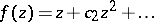that are holomorphic and univalent in:where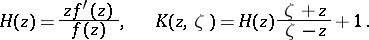Here,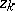(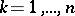;) are fixed points in,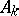are arbitrary complex constants, while, as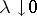, uniformly tends to zero with respect toon compact sets inside. In other words, in the class, which is not a linear space, for all functionsthere can be specified a one-parameter family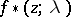,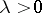, of functions of the same class such that on any closed set inthe expansion ofin powers ofis given by the above formula. Similar formulas (with an estimate of the order of smallness of the residual term on compact sets inside the respective domain) are also valid for other classes of analytic functions.

A characteristic feature of the method of internal variations is that differential equations for boundary or extremal functions may be obtained from the variational formulas. The study of such equations, involving the use of the analytical theory of differential equations, yields important qualitative results, and in a number of cases a complete solution of the extremal problem may be obtained.

The method has been successfully employed in problems on non-overlapping domains, and has become a constituent part of the so-called variation-parametric method .

How to Cite This Entry:
Internal variations, method of. I.A. Aleksandrov (originator), Encyclopedia of Mathematics. URL: http://encyclopediaofmath.org/index.php?title=Internal_variations,_method_of&oldid=18190
This text originally appeared in Encyclopedia of Mathematics - ISBN 1402006098